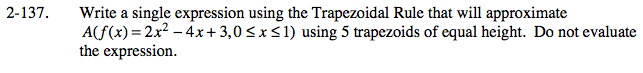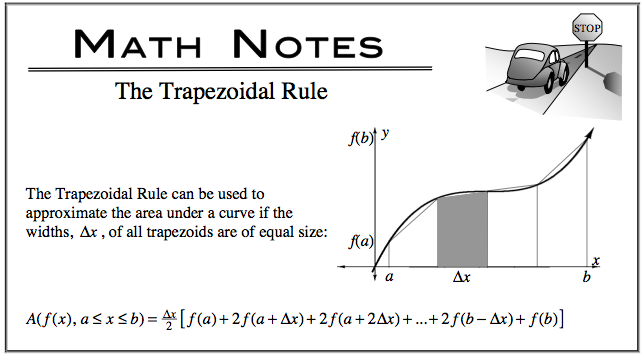### Home > CALC > Chapter Ch2 > Lesson 2.4.1 > Problem2-137

2-137.

Write a single expression using the Trapezoidal Rule that will approximate A(f(x) = 2x2 − 4x + 3, 0 ≤ x ≤ 1) using 5 trapezoids of equal height. Do not evaluate the expression. Homework Help ✎$\text{You know that you're writing an expression between }0\leq x\leq 1 \text{ using }5 \text{ trapezoids,}$

$\text{so you know that you'll be starting with }0 \text{ and increasing }x-\text{values by increments of }\frac{1}{5}$

$\text{until you've created }5\text{ trapezoids.}$